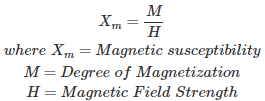# In a load commutated DC – DC chopper, the capacitor has a_____________?

A. Symmetric triangular voltage across itself
B. Symmetric rectangular voltage across itself
C. Symmetric trapezoidal voltage across itself
D. Symmetric sinusoidal voltage across itself…

# Which of the following quantities consists of SI unit as WATT ?

A. Force
B. Charge
C. Current
D. Power

The SI unit of power is the watt and it is discover by the Scottish Engineer James Watt. Power is the rate of consumption of electric energy. So, it is equivalent to joules per second.…

# Which of the following has no units?

A. Permeability
B. Moment of a magnet
C. Magnetic susceptibility
D Permittivity

Magnetic susceptibility is the degree to which a material is magnetized by an external magnetic field, hence in the SI system it is dimensionless.A. Energy
B. Time
C. Power
D. Charge

A. Current
B. Voltage
C. Power
D. Speed

A. zero
B. infinite
C. finite
B. 100 ohms

A. zero
B. infinite
C. finite
D. 100 ohms

# Capacitor does not allow the sudden change of ?

A. current
B. voltage
C. power
D. None of the above

# Power dissipation in ideal inductor is

A. Maximum
B. Minimum
C. Zero
D. A finite value

A. 10
B. 60
C. 600
D. 1200

A. Ω.
B. Ω – metre.
C. Ω / metre.
D. Ω / m².